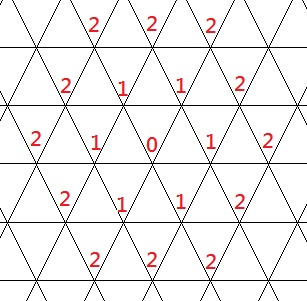Problems

# Triangular web

Time limit 1 second
Memory limit 128 MiB

In front of you there is an endless triangular grid. It is arranged in such a way that if you fire a vertex, then this vertex lights up, next second all the vertices next to the given one light up, then all the vertices next to those already burning light up, etc. Consider that the fire will never go out.

Initially, only one vertex fires. Find the number of burning vertices after n seconds.## Input data

One number n (0n10^9).

## Output data

Print the number of burning vertices after n seconds.

## Examples

Input example #1
1

Output example #1
7

Input example #2
1500

Output example #2
6754501

Author Andrej Selivanov
Source "Five for week" 05 (2013-2014), Breadth First Search